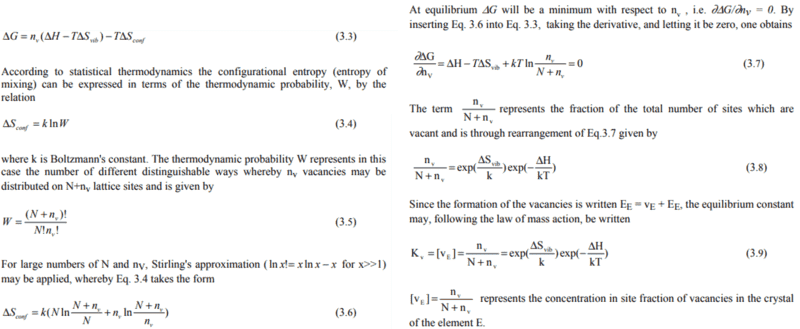# Defect concentration formula w/o Stirling approximation

TL;DR Summary
The defect concentration is normally expressed by using Stirling approximation (SA) for very nice simplicity. However, in the case of wide bandgap materials, it is common to see the concentrations of electrons or defects are too small to use SA. Could you give me some nice ideas to express the low concentration of species, which can be lower than 1 cm^-3.
In many cases, the concentrations of defects or charges are quite big enough to use SA, due to a big number of Avogadro's number.
The derivation for the well-known formula of a defect concentration is followed.If the n_v is expected to be lower than 1, then it would be impossible to use SA.
Then, how can we know the exact concentration of the defect?
I tried to use the gamma function, however, it behaves wield at the region lower than 1.
Thank you for reading this post.